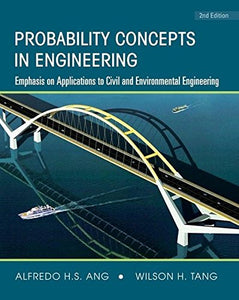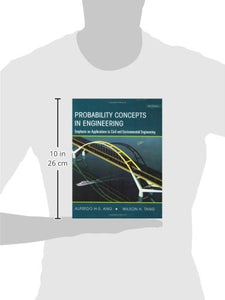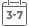# Probability Concepts In Engineering: Emphasis On Applications To Civil And Environmental Engineering (V. 1)

• Publish Date: 2006-03-03
• Binding: Hardcover
Vendor
Wiley
Regular price
\$234.50
Sale price
\$88.00
Quantity must be 1 or more

Attention: For textbook, access codes and supplements are not guaranteed with used items.Apply the principles of probability and statistics to realistic engineering problems

The easiest and most effective way to learn the principles of probabilistic modeling and statistical inference is to apply those principles to a variety of applications. That's why Ang and Tang's Second Edition of Probability Concepts in Engineering (previously titled Probability Concepts in Engineering Planning and Design) explains concepts and methods using a wide range of problems related to engineering and the physical sciences, particularly civil and environmental engineering.

Now extensively revised with new illustrative problems and new and expanded topics, this Second Edition will help you develop a thorough understanding of probability and statistics and the ability to formulate and solve real-world problems in engineering. The authors present each basic principle using different examples, and give you the opportunity to enhance your understanding with practice problems. The text is ideally suited for students, as well as those wishing to learn and apply the principles and tools of statistics and probability through self-study.

Key Features in this 2nd Edition:
* A new chapter (Chapter 5) covers Computer-Based Numerical and Simulation Methods in Probability, to extend and expand the analytical methods to more complex engineering problems.
* New and expanded coverage includes distribution of extreme values (Chapter 3), the Anderson-Darling method for goodness-of-fit test (Chapter 6), hypothesis testing (Chapter 6), the determination of confidence intervals in linear regression (Chapter 8), and Bayesian regression and correlation analyses (Chapter 9).
* Many new exercise problems in each chapter help you develop a working knowledge of concepts and methods.
* Provides a wide variety of examples, including many new to this edition, to help you learn and understand specific concepts.
* Illustrates the formulation and solution of engineering-type probabilistic problems through computer-based methods, including developing computer codes using commercial software such as MATLAB and MATHCAD.
* Introduces and develops analytical probabilistic models and shows how to formulate engineering problems under uncertainty, and provides the fundamentals for quantitative risk assessment.